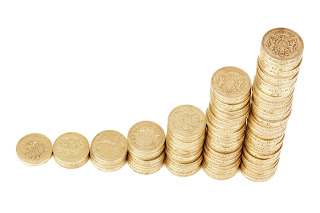Skip to main content

### Economic growth vs Economic development

Economic growth, is an increase in the level of output of goods and services measured in terms of value added thus we can say Economic growth is a narrow concept while Economic development is the progressive change in the socio-economic structure of the economy thus Economic development is a broader concept.
Economic growth is only a quantitatively concept, it means we can study or read the data mathematically but Economic development is the quantitatively as well as qualitatively concept means that it's not necessary that we can read data mathematically for example improvement in the Education.
Economic development accounts for the structural , institutional and technical change in the economy (the economy will move from primary oriented to secondary and tertiary oriented sector) thus there is a shift in labor force from primary sector to secondary and tertiary sector but for Economic growth it is not necessarily the case.
Economic growth ignores the distribution of the income in the economy. in economic growth there is a possibility of rising income inequality in the nation while under Economic development it evenly distribute income and it make sure that income inequality does not rise.Add caption

Economic growth affect environment adversely it means that there is  increase in the pollution level but Economic development doesn't affect environmentEconomic growth vs economic development

### Solow growth model assumptions of solow growth model and steady state in solow model

The SolowGrowth model is an exogenous model of economic Growth  that analyzes changes in the level of output in an economy over time as a result of changes in the population growth rate, the savings rate, and the rate of technological progress.

Assumptions of the Solow growth model:
1. One composite good produced which is either consumed or accumulated in capital form
2. Homogeneous labour
3. Two factors of production— Capital (K) and Labour
4. Constant Return to Scale (CRS) production function
5. Economy exhibits diminishing returns in the labor and capital inputs.
6. Marginal Propensity to Save (s)—assumed constant
7. Exogenously given labour force growth rate (n)
8. Closed economy and Laissez Faire

Structure of the solow growth model:

Suppose that there is no depreciation, Consider aggregate production function.
Y=F(K,L) Where Y is the aggregate output K is the aggregate capital and L is the labour force
We have already assumed that production function exhibit constant returns to scale that mean…

### Harrod Domar model, assumptions of Harrod Domar model, it's working and limitations of Harrod Domar model

Harrod domar model is an economic growth model, the model suggest that economic growth rates depend upon two things savings and capital output ratio. Higher savings enables higher investment thus higher growth rate , while lower-output ratio means Investment is more efficient thus the growth rate will be higher.
Mathematically growth rate (g) α level of savings  growth rate (g) α 1/capital output ratio(k)

Assumtions of the Harrod Model- 1. Savings leads to investment S= I
2. Investment leads to change in capital stocks I= ΔK
3. Constant capital output ratio (r) = K/Y.
4. There is no government intervention it the functioning of the economy.
5. A full- employment level of income already exist.
6. There are no lags in adjustment of variables.
7. Average propensity to save (aps) equals to marginal propensity      to save (mps) that means absolute change equals to relative change.
Harrod model is depends upon three growth rates a) Actual growth rates (G). b) Wwarranted growth rates (Gw).
c) Natural gr…

### Balance of payments (BOP)

Balance of Payments (BOPs) is the record of all trade and financial transactions of one country to the rest of world. The direction of money flow determines whether a particular item is Credit or Debit. For example export of a good is credit (since your country will receive money in exchange of goods) and foreign investment is a credit, similarly import of goods and investment abroad is a debit.

BOP has two Accounts - Current Account and Capital Account, All current or revenue expenditure transaction (such as export import transfer payment, non-factor payments) are recorded in current Account. Transactions that influence country’s Capital asset are recorded in Capital Account.

BOP equilibrium is achieved when the sum of current Account balance and Capital Account balance equal to zero, If addition of these two Accounts results in a surplus (deficit) then it is indicated as BOP surplus (deficit).